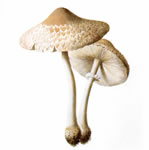# Mushroom

## Donated on 4/26/1987

From Audobon Society Field Guide; mushrooms described in terms of physical characteristics; classification: poisonous or edible

Multivariate

Life Science

Classification

Categorical

8124

22

# Dataset Information

This data set includes descriptions of hypothetical samples corresponding to 23 species of gilled mushrooms in the Agaricus and Lepiota Family (pp. 500-525). Each species is identified as definitely edible, definitely poisonous, or of unknown edibility and not recommended. This latter class was combined with the poisonous one. The Guide clearly states that there is no simple rule for determining the edibility of a mushroom; no rule like ``leaflets three, let it be'' for Poisonous Oak and Ivy.

Has Missing Values?

Yes

# Variables Table

Variable NameRoleTypeDemographicDescriptionUnitsMissing Values
poisonousTargetCategoricalno
cap-shapeFeatureCategoricalbell=b,conical=c,convex=x,flat=f, knobbed=k,sunken=sno
cap-surfaceFeatureCategoricalfibrous=f,grooves=g,scaly=y,smooth=sno
cap-colorFeatureBinarybrown=n,buff=b,cinnamon=c,gray=g,green=r, pink=p,purple=u,red=e,white=w,yellow=yno
bruisesFeatureCategoricalbruises=t,no=fno
odorFeatureCategoricalalmond=a,anise=l,creosote=c,fishy=y,foul=f, musty=m,none=n,pungent=p,spicy=sno
gill-attachmentFeatureCategoricalattached=a,descending=d,free=f,notched=nno
gill-spacingFeatureCategoricalclose=c,crowded=w,distant=dno
gill-colorFeatureCategoricalblack=k,brown=n,buff=b,chocolate=h,gray=g, green=r,orange=o,pink=p,purple=u,red=e, white=w,yellow=yno

0 to 10 of 23

1. cap-shape: bell=b,conical=c,convex=x,flat=f, knobbed=k,sunken=s 2. cap-surface: fibrous=f,grooves=g,scaly=y,smooth=s 3. cap-color: brown=n,buff=b,cinnamon=c,gray=g,green=r, pink=p,purple=u,red=e,white=w,yellow=y 4. bruises?: bruises=t,no=f 5. odor: almond=a,anise=l,creosote=c,fishy=y,foul=f, musty=m,none=n,pungent=p,spicy=s 6. gill-attachment: attached=a,descending=d,free=f,notched=n 7. gill-spacing: close=c,crowded=w,distant=d 8. gill-size: broad=b,narrow=n 9. gill-color: black=k,brown=n,buff=b,chocolate=h,gray=g, green=r,orange=o,pink=p,purple=u,red=e, white=w,yellow=y 10. stalk-shape: enlarging=e,tapering=t 11. stalk-root: bulbous=b,club=c,cup=u,equal=e, rhizomorphs=z,rooted=r,missing=? 12. stalk-surface-above-ring: fibrous=f,scaly=y,silky=k,smooth=s 13. stalk-surface-below-ring: fibrous=f,scaly=y,silky=k,smooth=s 14. stalk-color-above-ring: brown=n,buff=b,cinnamon=c,gray=g,orange=o, pink=p,red=e,white=w,yellow=y 15. stalk-color-below-ring: brown=n,buff=b,cinnamon=c,gray=g,orange=o, pink=p,red=e,white=w,yellow=y 16. veil-type: partial=p,universal=u 17. veil-color: brown=n,orange=o,white=w,yellow=y 18. ring-number: none=n,one=o,two=t 19. ring-type: cobwebby=c,evanescent=e,flaring=f,large=l, none=n,pendant=p,sheathing=s,zone=z 20. spore-print-color: black=k,brown=n,buff=b,chocolate=h,green=r, orange=o,purple=u,white=w,yellow=y 21. population: abundant=a,clustered=c,numerous=n, scattered=s,several=v,solitary=y 22. habitat: grasses=g,leaves=l,meadows=m,paths=p, urban=u,waste=w,woods=d

Class Labels

edible=e, poisonous=p

# Papers Citing this Dataset

topFiberM: Scalable and Efficient Boolean Matrix Factorization

By Abdelmoneim Desouki, Michael Roder, Axel-Cyrille Ngomo. 2019

Published in ArXiv.

Estimating Risk and Uncertainty in Deep Reinforcement Learning

By William Clements, Benoit-Marie Robaglia, Bastien Delft, Reda Slaoui, S'ebastien Toth. 2019

Published in ArXiv.

High-Performance Support Vector Machines and Its Applications

By Taiping He, Tao Wang, Ralph Abbey, Joshua Griffin. 2019

Published in ArXiv.

Bayesian Learning of Neural Network Architectures

By Georgi Dikov, Patrick Smagt, Justin Bayer. 2019

Published in ArXiv.

Edibility Detection of Mushroom Using Ensemble Methods

By Nusrat Pinky, S. Islam, Rafia Alice. 2019

Published in International Journal of Image, Graphics and Signal Processing.

0 to 5 of 77

77 citations
100498 views

ecology